Courses

Test: Mechanical Properties of Materials - 1

10 Questions MCQ Test Solid Mechanics | Test: Mechanical Properties of Materials - 1

Description
Attempt Test: Mechanical Properties of Materials - 1 | 10 questions in 30 minutes | Mock test for Mechanical Engineering preparation | Free important questions MCQ to study Solid Mechanics for Mechanical Engineering Exam | Download free PDF with solutions
QUESTION: 1

Strain is defined as ratio of

Solution:

The strain is defined as the ratio of change in dimension to the original dimension.

If ‘δl’ changes in the length and ‘l’ is the original length, strain = δl/l

Important Terms:

• The ratio of change in length to the original length is called the longitudinal strain.
• The ratio of change in diameter/breadth to original diameter/breadth is called the lateral strain.
• The volumetric strain of a deformed body is defined as the ratio of the change in volume of the body to the deformation to its original volume.
• Shear strain is defined as the strain accompanying a shearing action. It is the angle in radian measure through which the body gets distorted when subjected to external shearing action.
QUESTION: 2

Solution:
QUESTION: 3

Young's modulus is defined as the ratio of

Solution:

The linear relationship between stress and strain for a bar in simple tension or compression can be expressed by the equation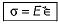in which Eis a constant of proportionality known as modulus of elasticity for the material. The modulus of elasticity is the slope of the stress- strain diagram in the linearly elastic region and its value depends upon the particular material being used.

QUESTION: 4

Match List-I (Material) with List-ll (Young’s modulus):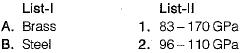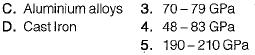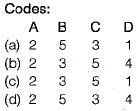Solution: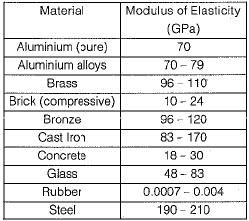QUESTION: 5

The permanent mode of deformation of a material known as ____________

Solution:

Plasticity is defined as the property of a material due to which it is permanently deformed due to loading. Elasticity is the temporary form of deformation. Twinning and Slip are mechanisms of Plastic deformation.

QUESTION: 6

A thin mid steel wire is loaded by adding loads in equal increments till it breaks. The extensions noted with increasing loads will behave as under

Solution:

At first, the strain is proportional to strain or elongation is proportional to the load giving a straight-line relationship.

A further increase in the load after yield load will cause marked deformation in the whole volume of the metal. The maximum load which the specimen can withstand without failure is called the load at the ultimate strength.

QUESTION: 7

Match List-1 (Materia!) with List-ll (Poisson’s Ratio)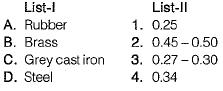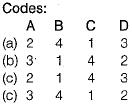Solution:
QUESTION: 8

During a tensile test on a specimen of 1 cm2 cross-section, maximum load observed was 80 kN and area of cross-section at neck was 0.5 cm2. UTS of specimen is

Solution: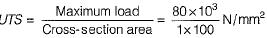= 800 MPa

QUESTION: 9

The ability of a material to resist plastic deformation known as _____________

Solution:

The point of stretching where it increases suddenly is known as yield strength, i.e. the region where the stretch is elastic. Tensile strength is the force needed to fracture the material. Impact strength is the capacity of a material to resist shock energy before a fracture.

QUESTION: 10

Resilience of a material is considered when it is subjected to

Solution:

Resilience represent the ability of the material to absorb energy within the elastic range.Use Code STAYHOME200 and get INR 200 additional OFF Use Coupon Code

Track your progress, build streaks, highlight & save important lessons and more!

Similar Content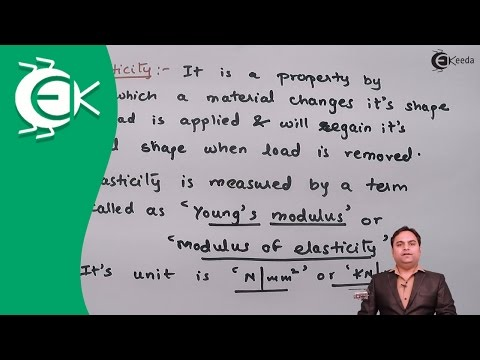Related tests